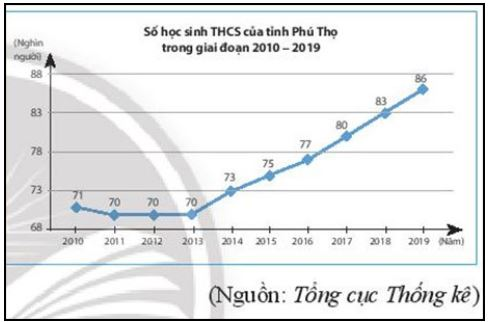## Solve exercises at the end of chapter 9 – Math 7 Horizons – Math Book

Solve exercises at the end of chapter 9 – Math 7 Horizons
==========

### Solution 1 page 96 Math textbook 7 Creative horizon volume 2 – CTST

There are 3 comic books and 1 textbook on the bookshelf. An randomly selects 2 books from the bookshelf. Which of the following events is certain, unlikely, or random. Why?

A: “An chooses 2 comic books”.

B: “An chooses at least 1 comic book”.

C: “An chooses 2 textbooks”.

Detailed instructions for solving Lesson 1

Solution method

We rely on the correctness of the event and then consider it as a sure, impossible or random event

Detailed explanation

Event A is a random event because it is possible for An to choose 2 comic books but also 1 comic book and 1 textbook.

Event B is a sure event because An taking 2 books at random will always get 1 comic book

Event C is an impossible event because there is only one textbook on the shelf.

–>

— *****

### Solution 2 page 96 Math textbook 7 Creative horizon volume 2 – CTST

Roll two balanced dice. Compare the probabilities of the following events:

A: “The total number of dots appearing on the top of the two dice is even”,

B: “The number of dots appearing on the top two dice is 6”,

C: “The number of dots appearing on the top of two dice is equal”.

Detailed instructions for solving Lesson 2

Solution method

We calculate the probability of events A, B, C and then compare the events

Detailed explanation

Event C: the same number of dots on 2 faces will have an even result, but since event C requires 2 dice rolled with the same number of dots, event A only needs the sum of the dots on the 2 dice to be even So if 1 dice rolls 3 and 1 dice rolls out 1, event A still occurs. So P(C) < P(A)

Event B : the number of dots on both sides is 6 (total is 12 dots) with probability $$\frac{1}{{11}}$$ and there is only 1 outcome so P(B) will be correct. lowest capacity.

–>

— *****

### Solution 3 page 96 Math textbook 7 Creative horizon volume 2 – CTST

A box has 4 cards of the same size and are numbered 2, 4, 6, 8 respectively. Pick 1 card at random from the box. Calculate the probabilities of the following events:

A: “Get a card with a prime number”

B: “Get an odd number card”

C: “Get an even number card

Detailed instructions for solving Lesson 3

Solution method

We consider the probabilities of the events and then compute the probabilities

Detailed explanation

Event A is a random event because 2 is a prime number.

The number of possible outcomes is 4. The number of possibilities for event A is 1 (get card number 2)

$$\Rightarrow P(A)=\dfrac{1}{4}$$

Since none of the cards have an odd number, B is an impossible event, so P(B) = 0

Since all cards are even, C is a sure event $$\Rightarrow P(C) = 1$$

–>

— *****

### Solution 4 page 96 Math textbook 7 Creative horizon volume 2 – CTST

A closed box contains 5 balls of equal size and mass, of which 1 are blue and 4 are red. Pick a ball at random from the box, calculate the probability of the following events

A: “The ball taken out is yellow”

B: “The ball taken out is blue”.

Detailed instructions for solving Lesson 4

Solution method

We calculate the number of outcomes (n) and then calculate the probability of the events occurring.

Detailed explanation

Since there is no yellow ball in the box, P(A) = 0

The number of results is 1 + 4 = 5.

The number of chances that event B will occur is 1.

$$\Rightarrow P(B) = \dfrac{1}{5}$$

–>

— *****

### Solve problems 5 pages 96 Math textbook 7 Creative horizon volume 2 – CTST

The chart below shows the number of junior high school students in Phu Tho province from 2010 to 2019.Randomly select a year in that period. Know the probability of choosing each year is the same

a) State the set of possible outcomes for the selected year.

b) Let B be the event: “Phu Tho province has over 85,000 junior high school students in the selected year”. Calculate the probability of the event.

Detailed instructions for solving Lesson 5

Solution method

a) We find all possible years and write down all possible outcomes

b) We calculate the number of possible outcomes, the number of possibilities of event B, and then find P(B)

Detailed explanation

a) Possible outcomes are: {2010;2011;2012;2013;2014;2015;2016;2017;2018;2019}

b) Number of possible outcomes n = 10.

The number of chances that event B will occur is: 1

$$\Rightarrow P(B) =\dfrac{1}{{10}}$$

–>

— *****